Courses

# Test: Seepage Analysis - 1

## 10 Questions MCQ Test GATE Civil Engineering (CE) 2022 Mock Test Series | Test: Seepage Analysis - 1

Description
This mock test of Test: Seepage Analysis - 1 for Civil Engineering (CE) helps you for every Civil Engineering (CE) entrance exam. This contains 10 Multiple Choice Questions for Civil Engineering (CE) Test: Seepage Analysis - 1 (mcq) to study with solutions a complete question bank. The solved questions answers in this Test: Seepage Analysis - 1 quiz give you a good mix of easy questions and tough questions. Civil Engineering (CE) students definitely take this Test: Seepage Analysis - 1 exercise for a better result in the exam. You can find other Test: Seepage Analysis - 1 extra questions, long questions & short questions for Civil Engineering (CE) on EduRev as well by searching above.
QUESTION: 1

### From a flow net which of the following information can be obtained? 1. Rate of flow 2. Pore water pressure 3. Exit gradient 4. Permeability Select the correct answer using the codes given below:

Solution:

Seepage pressures, uplift pressures, exit gradient and pore-water pressure can be obtained from a flownet.

QUESTION: 2

Solution:
QUESTION: 3

### A flownet of a coffer dam foundation has 6 flow channels and 18 equipotential drops. The head of water lost during seepage is 6 m. If the coefficient of permeability of foundation is 4 x 10-5 m/min, then the seepage loss per metre length of dam will be

Solution:

Seepage loss per metre length,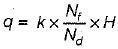Given, k = 4 x 10-5 m/min
= 4 x 10-5 x 24 x 60 m/day
= 0.0576 m/day
∴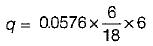= 0.1152 m3/day
= 11.52 x 10-2 m3/day
Nf is number of flow channels = 6.0
Nd is number of equipotential drops = 18

QUESTION: 4

Capillary rise is maximum for

Solution: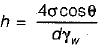For fine grained soils effective size ‘d’ is smaller so it will experience more capillary rise.

QUESTION: 5

Water is flowing in an upward direction through a stratum of sand, 4 m thick, under a total head difference of 2 m. The sand has a specific gr. of 2.65 and void ratio of 0.065. The factor of safety against quick sand condition would be

Solution: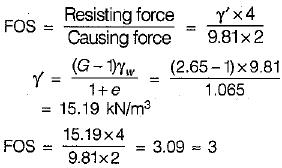QUESTION: 6

For design of filter the criteria usually adopted is

Solution: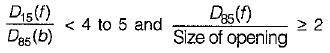QUESTION: 7

The constant of proportionality between seepage velocity and hydraulic gradient is called

Solution:

Vs = kpi
Where, Vs = seepage velocity
kp = coeff. of percolation
Seepage Velocity = Coefficient of Percolation x Hydraulic Gradient

QUESTION: 8

In an earthen dam the phreatic line is

Solution:
QUESTION: 9

The critical hydraulic gradient of a soil ic is given by
where G = specific gravity, e = void ratio

Solution: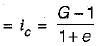also,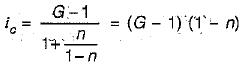QUESTION: 10

If the permeability of soil does not depend on its mineral content but depends on orientation of particles then the void space is known as

Solution:

Micropores, often found between and within soil aggregates, are so small that surface tension holds water in place. It does not depend on mineral content rather depends on orientation of the particles. Instead of draining away, water moves through micropores only when suction is created by thirsty roots.